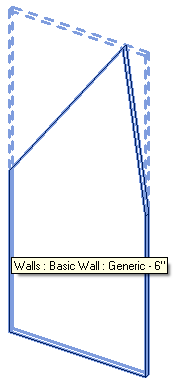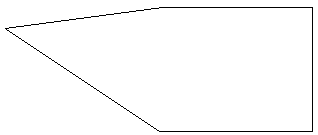### Planar Face Transform

Here is another contribution by Joe Offord of Enclos, who already shared valuable insights on accessing curtain wall geometry, speeding up the interactive selection process, mirroring in a new family and changing the active view.

Above all, he implements all his stuff in VB, so this is also something for you VB aficionados.

This time, Joe looks at a command that redraws a planar face's edges in a drafting view. The issue is somewhat related to the discussion of polygon transformations.

However, instead of using rotations and translations, which can be difficult to determine in 3D, Joe wrote a utility function that re-maps the global coordinate system to the planar face's coordinate system using vectors and origins.

The command prompts the user to select a planar face on an elements, creates a new drafting view, retrieves the edges of the selected face, and transforms them from the 3D space to the drafting view.

To test this, I selected the following slightly lopsided wall in 3D:The command generated a new drafting view showing the wall profile edges like this:Here is the Execute method implementation for the mainline of the command:

```  Public Function Execute( _
ByVal commandData As ExternalCommandData, _
ByRef message As String, _
ByVal elements As ElementSet) As Result Implements IExternalCommand.Execute

Dim uiapp As UIApplication = commandData.Application
Dim app As Application = uiapp.Application
Dim uidoc As UIDocument = uiapp.ActiveUIDocument
Dim doc As Document = uidoc.Document
Dim sel As Selection = uidoc.Selection

Try
Dim ref As Reference = sel.PickObject( _
ObjectType.Face, "Select a face")

Dim elem As Element = doc.GetElement(ref)

Dim gObj As GeometryObject _
= elem.GetGeometryObjectFromReference(ref)

Dim face As PlanarFace = TryCast(gObj, PlanarFace)

If face Is Nothing Then
MsgBox("Not a planar face")
End If

Dim v As ViewDrafting = Nothing

Dim tr As New Transaction(doc, "Draw Face")
tr.Start()

Try
v = doc.Create.NewViewDrafting 'create a new view
v.Scale = 48 '1/4" = 1'-0"

'this transform re-orients the global
'coordinate system to the face's coordinate system
Dim trans As Transform _
= Util.PlanarFaceTransform(face)

For Each eArr As EdgeArray In face.EdgeLoops
For Each e As Edge In eArr
Dim c As Curve = e.AsCurveFollowingFace(face)
c = c.Transformed(trans) 'orient the curve on the XY plane
doc.Create.NewDetailCurve(v, c) 'draw the curve
Next
Next

tr.Commit()
Catch ex As Exception
tr.RollBack()
MsgBox("Error: " + ex.Message)
End Try

If tr.GetStatus = TransactionStatus.Committed Then
uidoc.ActiveView = v
End If

Catch ex As Exception
MsgBox("Error: " + ex.Message)
End Try

Return Result.Succeeded

End Function
```

The transformation from the planar face coordinate system is created by the following two utility methods:

```  ''' <summary>
''' Return a transform that changes a x,y,z
''' coordinate system to a new x',y',z' system
''' </summary>
Public Shared Function TransformByVectors( _
ByVal oldX As XYZ, _
ByVal oldY As XYZ, _
ByVal oldZ As XYZ, _
ByVal oldOrigin As XYZ, _
ByVal newX As XYZ, _
ByVal newY As XYZ, _
ByVal newZ As XYZ, _
ByVal newOrigin As XYZ) As Transform

' [new vector] = [transform]*[old vector]
' [3x1] = [3x4] * [4x1]
'
' [v'x]   [ i*i'  j*i'  k*i'  translationX' ]   [vx]
' [v'y] = [ i*j'  j*j'  k*j'  translationY' ] * [vy]
' [v'z]   [ i*k'  j*k'  k*k'  translationZ' ]   [vz]
'                                               [1 ]
Dim t As Transform = Transform.Identity

Dim xx As Double = oldX.DotProduct(newX)
Dim xy As Double = oldX.DotProduct(newY)
Dim xz As Double = oldX.DotProduct(newZ)

Dim yx As Double = oldY.DotProduct(newX)
Dim yy As Double = oldY.DotProduct(newY)
Dim yz As Double = oldY.DotProduct(newZ)

Dim zx As Double = oldZ.DotProduct(newX)
Dim zy As Double = oldZ.DotProduct(newY)
Dim zz As Double = oldZ.DotProduct(newZ)

t.BasisX = New XYZ(xx, xy, xz)
t.BasisY = New XYZ(yx, yy, yz)
t.BasisZ = New XYZ(zx, zy, zz)

' The movement of the origin point
' in the old coordinate system

Dim translation As XYZ = newOrigin - oldOrigin

' Convert the translation into coordinates
' in the new coordinate system

Dim translationNewX As Double _
= xx * translation.X _
+ yx * translation.Y _
+ zx * translation.Z

Dim translationNewY As Double _
= xy * translation.X _
+ yy * translation.Y _
+ zy * translation.Z

Dim translationNewZ As Double _
= xz * translation.X _
+ yz * translation.Y _
+ zz * translation.Z

t.Origin = New XYZ( _
-translationNewX, _
-translationNewY, _
-translationNewZ)

Return t

End Function

Public Shared Function PlanarFaceTransform( _
ByVal face As PlanarFace) As Transform

Return Util.TransformByVectors( _
XYZ.BasisX, _
XYZ.BasisY, _
XYZ.BasisZ, _
XYZ.Zero, _
face.Vector(0), _
face.Vector(1), _
face.Normal, _
face.Origin)
End Function
```

Here is PlanarFaceTransform.zip including the complete source code and Visual Studio solution of this command.

Many thanks to Joe for sharing this!